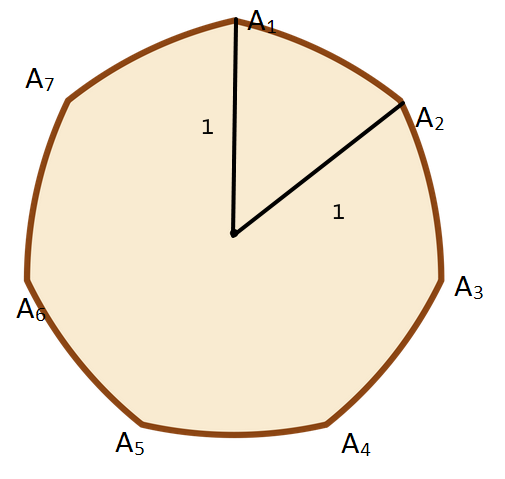# This world needs more coin of such shape!The diagram above shows a Reuleaux septagon drawn from a regular septagon with circumradius 1, where arc $A_1 A_2$ is drawn from center $A_5$, arc $A_2 A_3$ is drawn from center $A_6$, arc $A_3 A_4$ is drawn from center $A_7$, and so on.

Find the area of the Reuleaux septagon to 5 decimal places.

Bonus: Generalize this for all Reuleaux $n$-gon where $n$ is an odd number.

Inspiration

×